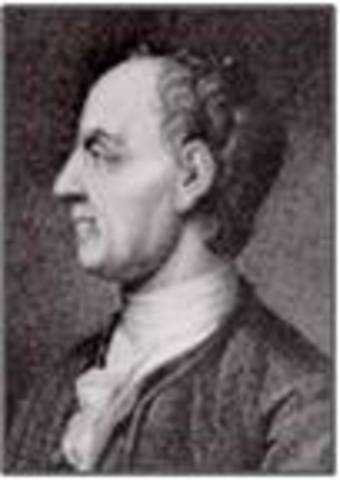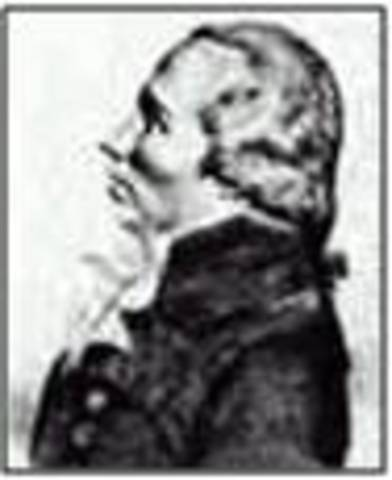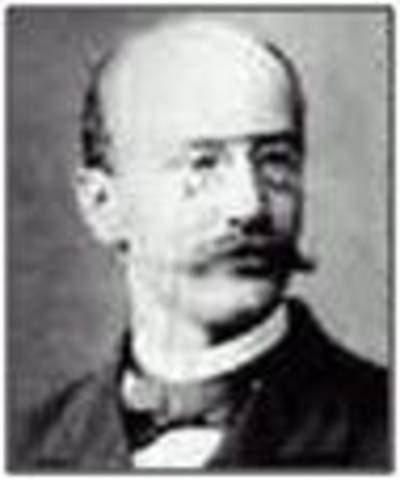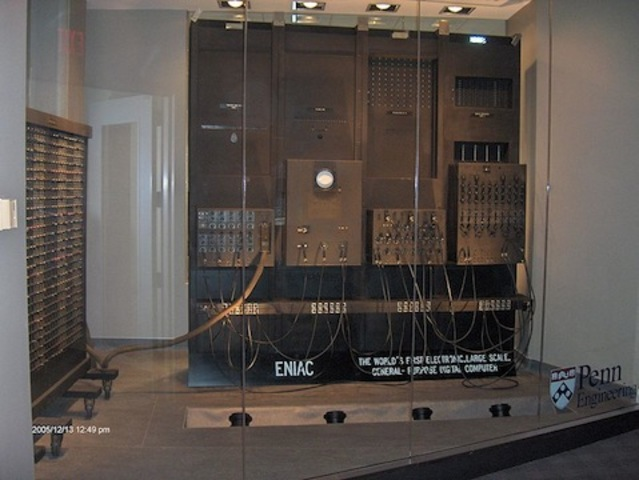• Ludoplh van Cuelen calculates π to 35 digitsLudolph van Ceulen spent a major part of his life calculating the numerical value of the mathematical constant π, using essentially the same methods as those employed by Archimedes some seventeen hundred years earlier. After his death, the "Ludolphine number", 3.14159265358979323846264338327950288...,
was engraved on his tombstone in Leiden.
• John Machin calculates π to 100 decimal placesCalculated all by hand, it took him months!
• Symbol π introduced by Leonard EulerAlthough used first by William Jones in 1706 (short for “periphery”), he did not have the weight to make it popular. Once the renowned Euler (“Oiler”) picked it up (previously using “p” or “c”) it became the standard.
• Johann Lambert proved π irrationalFirst, he proved –
If x is rational, (x ≠0), then tan x cannot be rational.
i.e., If tan x is rational, then x must be irrational or 0.
Therefore, Since tan π/4 = 1, π/4 must be irrational.
Q.E.D.
• Adrien-Marie Legendre proved π2 irrationalIrrational - Real but not expressible as the quotient of two integers
• Joseph Liouville proved transcendental numbers exist(used limits of continued fractions) Transcendental - it is not a root of a non-constant polynomial equation with rational coefficients.
• Charles Hermite proved e transcendentalThe mathematical constant e is the unique real number such that the value of the derivative (slope of the tangent line) of the function f(x) = ex at the point x = 0 is equal to 1
• William Shanks calculates π to 707 decimals

Later Ferguson finds an error in the 527th onward
• π-Day, (and Albert Einstein is born)• Ferdinand Lindemann proved π transcendental• D.F. Ferguson calculates π to 710 digits

Using a desktop calculator
• ENIAC computer (DoD & U. of Pen.) finds π to 2037 digitsThe advent of digital computers in the 20th century led to an increased rate of new π calculation records. John von Neumann et al. used ENIAC to compute 2037 digits of π in 1949, a calculation that took 70 hours
• CDC 7600 (Paris) calculates π to 1,000,000 digitsThis took 23 hours
• 1,000,000,000 digits of π calculated(USSR Chudnovsky brothers, NY)
• Hitachi SR8000 (Tokyo) calculated π to 206,158,430,000 digitsusing the Gauss-Legendre algorithm this took 37 hours